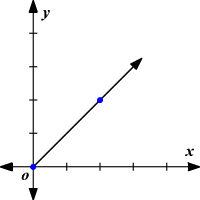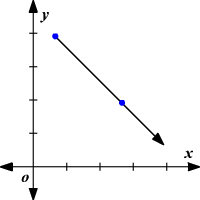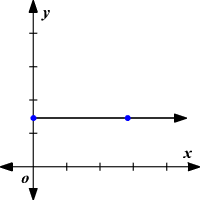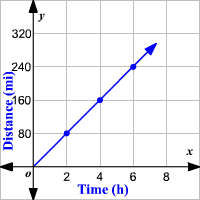# Rate of Change

A rate of change is a rate that describes how one quantity changes in relation to another quantity. If $x$ is the independent variable and $y$ is the dependent variable, then

$\text{rate}\text{\hspace{0.17em}}\text{\hspace{0.17em}}\text{of}\text{\hspace{0.17em}}\text{\hspace{0.17em}}\text{change}=\frac{\text{change}\text{\hspace{0.17em}}\text{\hspace{0.17em}}\text{in}\text{\hspace{0.17em}}\text{\hspace{0.17em}}y}{\text{change}\text{\hspace{0.17em}}\text{\hspace{0.17em}}\text{in}\text{\hspace{0.17em}}\text{\hspace{0.17em}}x}$

Rates of change can be positive or negative. This corresponds to an increase or decrease in the $y$ -value between the two data points. When a quantity does not change over time, it is called zero rate of change.

### Positive rate of change

When the value of $x$ increases, the value of $y$ increases and the graph slants upward.### Negative rate of change

When the value of $x$ increases, the value of $y$ decreases and the graph slants downward.### Zero rate of change

When the value of $x$ increases, the value of $y$ remains constant. That is, there is no change in $y$ value and the graph is a horizontal line .Example:

Use the table to find the rate of change. Then graph it.

$\begin{array}{|cc|}\hline \text{Time}\text{\hspace{0.17em}}\text{\hspace{0.17em}}\text{Driving}\text{\hspace{0.17em}}\text{\hspace{0.17em}}\left(\text{h}\right)\text{\hspace{0.17em}}x& \text{Distance}\text{\hspace{0.17em}}\text{\hspace{0.17em}}\text{Travelled}\text{\hspace{0.17em}}\left(\text{mi}\right)\text{\hspace{0.17em}}y\\ 2& 80\\ 4& 160\\ 6& 240\\ \hline\end{array}$

A rate of change is a rate that describes how one quantity changes in relation to another quantity.

$\begin{array}{l}\text{rate}\text{\hspace{0.17em}}\text{\hspace{0.17em}}\text{of}\text{\hspace{0.17em}}\text{\hspace{0.17em}}\text{change}=\frac{\text{change}\text{\hspace{0.17em}}\text{\hspace{0.17em}}\text{in}\text{\hspace{0.17em}}\text{\hspace{0.17em}}y}{\text{change}\text{\hspace{0.17em}}\text{\hspace{0.17em}}\text{in}\text{\hspace{0.17em}}\text{\hspace{0.17em}}x}\\ =\frac{\text{change}\text{\hspace{0.17em}}\text{\hspace{0.17em}}\text{in}\text{\hspace{0.17em}}\text{\hspace{0.17em}}\text{distance}}{\text{change}\text{\hspace{0.17em}}\text{\hspace{0.17em}}\text{in}\text{\hspace{0.17em}}\text{\hspace{0.17em}}\text{time}}\\ =\frac{160-80}{4-2}\\ =\frac{80}{2}\\ =\frac{40}{1}\end{array}$

The rate of change is $\frac{40}{1}$ or $40$ . This means a vehicle is traveling at a rate of $40$ miles per hour.